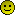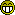Engine Management eCtune , Crome , AFC , FMU , Zdyne , Hondata , EMS , TE , Uberdata Anything to control your fuel, spark needs!

1.5 BAR

Join Date: Mar 2003
Posts: 1,190Q's about diy a/f meter

Hi everybody,

does anyone know what wattage resistors I should be using for the schematic at http://www.scirocco.org/tech/misc/afgauge/af.html? I know I need one 2.3kohm and 3.3kohm, but I don't know if it should be 1/2 watt or 1/4 watt.
thanks!0.5 BAR

Join Date: Apr 2003
Posts: 188Re:Q's about diy a/f meter

watt= volt x amp
amp= volt / resistence

teach a man to fish...1.0 BAR

Join Date: Sep 2003
Posts: 453Re:Q's about diy a/f meter

I'm casting, but nothing is biting. Could you or someone else elaborate on exactly how to USE your formulas in this instance?

I get the I=V/R, but I'm not sure how to use that to determine the appropriate wattage to use, or how much it matters. I slept thru most of electronics 101.0.0 BAR

Join Date: Jan 2004
Posts: 43Re:Q's about diy a/f meter

Originally Posted by d16tuner
I'm casting, but nothing is biting. Could you or someone else elaborate on exactly how to USE your formulas in this instance?

I get the I=V/R, but I'm not sure how to use that to determine the appropriate wattage to use, or how much it matters. I slept thru most of electronics 101.

How to use the formula I=V/R? Current (measured in 'amps') equals Voltage (measured in, erm, volts) divided by Resistance (measured in ohms). If you know the supplied voltage and the resistance of the load (ie. lightbuld, led, etc.) then you will find the amount of draw (ie. amps).

Not to speak to you as a simpleton, but, from I read of your post, this is what you were looking for?If not, welp.. <grin> ask a different way ;p1.0 BAR

Join Date: Sep 2003
Posts: 453Re:Q's about diy a/f meter

Duh! :P

I GET the relationship between Current, Voltage, and Resistance - that is one of the few things I DO remember from electronics. Altho I didn't know it before, I think I've even got a grasp on the second formula.

IE on a 12v system, with a 2.3k ohm resistor, you've got 5.2 mA. Plug that into the other formula and the resistor is drawing 62 mW.

I just don't understand how you can use that formula, and the other one to tell what wattage to use for the resistors. I need nelp with that next leap of logic.1.5 BAR

Join Date: Mar 2003
Posts: 1,190Re:Q's about diy a/f meter

I was going to post on the smartass reply after I read it the first time but I figured it wasn't worth itBut now I will, as I see I wasn't the only one who didn't get it on the first try. First you need to figure out the amperage. you do this by (amp=volt/resistance) taking 12 volts (cars electrical system) over resistance, say, 3.3k ohms. this gives you 0.00364 amps. then, to figure out how many watts you need the resistor to be able to handle, you take 12 volts times .00364 amps. gives you roughly 0.04 watts. so, a 1/4 watt resistor is more than enough for the job, and a 1/2 watt resistor is overkill.1.0 BAR

Join Date: Sep 2003
Posts: 453Re:Q's about diy a/f meter

Ok, so the rating on the resistor is the MAX that it will handle, and as long as it is lower than that you are ok, right? That is the part I didn't get, I guess - what the rating on the resistor is actually for - max, or if it needs to be between some values (say between 1/4 and 1/2). So in this case, the 1/4 Watt is ok, and anything over that would be ok too - they are just more robust?1.5 BAR

Join Date: Mar 2003
Posts: 1,190Re:Q's about diy a/f meter

yeah, just more robust I guess. But the 1/2 watt resistors are HUGE compared to the 1/4 watt resistors. plus the 1/4 watt resistors can handle about 10x more wattage than is going to be going through them... in this case there really wouldn't be a point to using 1/2 watt resistors.Forum
Replies
Last Post
Forced Induction
9
01-25-2011 05:45 PM
d16tuner
Engine Management
31
02-02-2004 10:55 PM
alldriver28
Turbo Parts For Sale
0
09-16-2003 01:10 PM
kokoro401
Forced Induction
2
08-20-2003 01:15 AM
DETROITREX
Forced Induction
3
02-15-2003 12:14 PMShow Printable VersionEmail this Page